Adding some values to the set

We have created the set now, and it is very easy. But now, we are more interested in adding some values to the set. We can do multiple things for this, and we are going to head towards it like we can give some iterable as input to the Set constructor, or we can make use of a method, called as add, to add some element to the set.

So, while creating the set, if we passed some iterable to the Set constructor, we are going to get those values in the set. For example, let’s consider the below code, in which, we are giving an array to the Set constructor –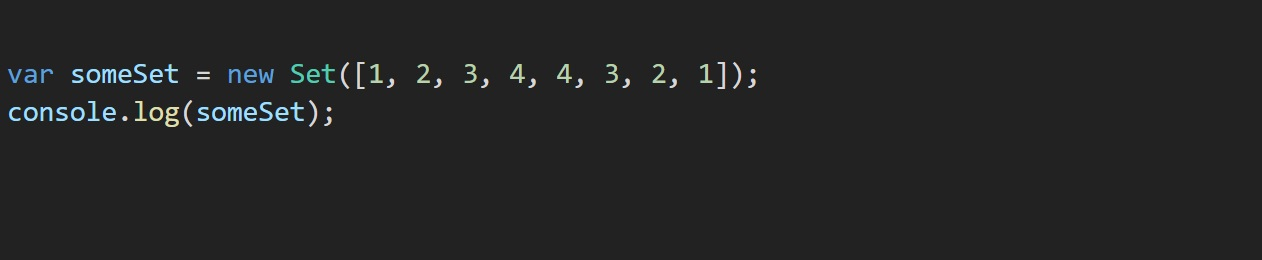As you can see, we have given an array to the Set constructor. But, the given array contains duplicate values, and we have seen that the set can have only unique values, so the duplicate values won’t be considered, and so, in the set, only the elements 1, 2, 3, and 4 would be added. Let’s have a look at the output now –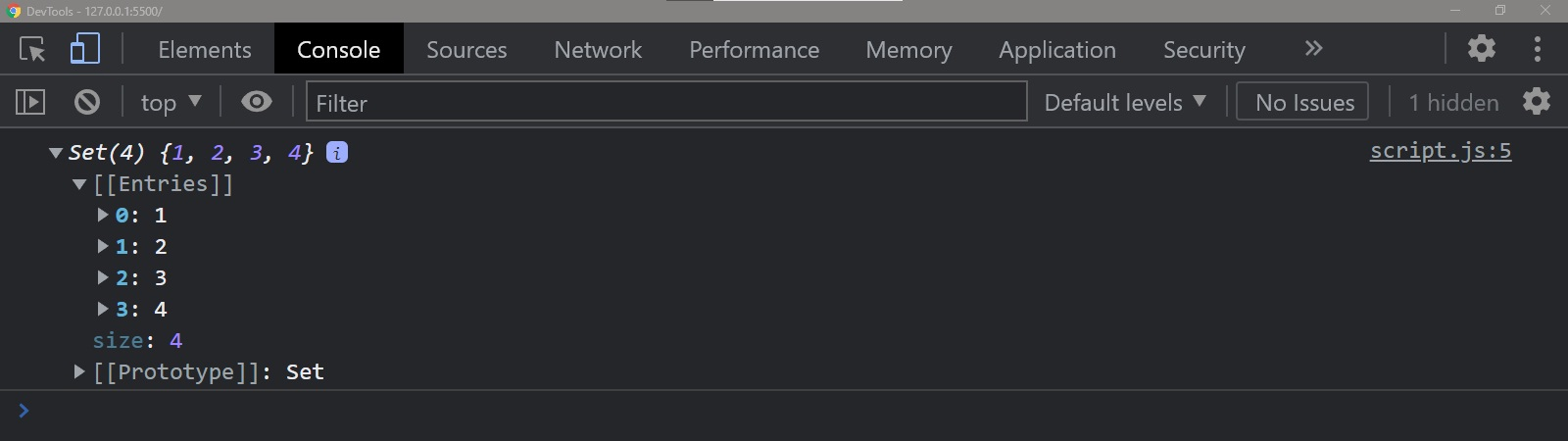As you can see, we got 4 elements into the set, namely 1, 2, 3, and 4. The other elements were repeated, so they were not added. There is one more way, to add some element to the set, which is, using the add method.

Let’s now try using the add method here –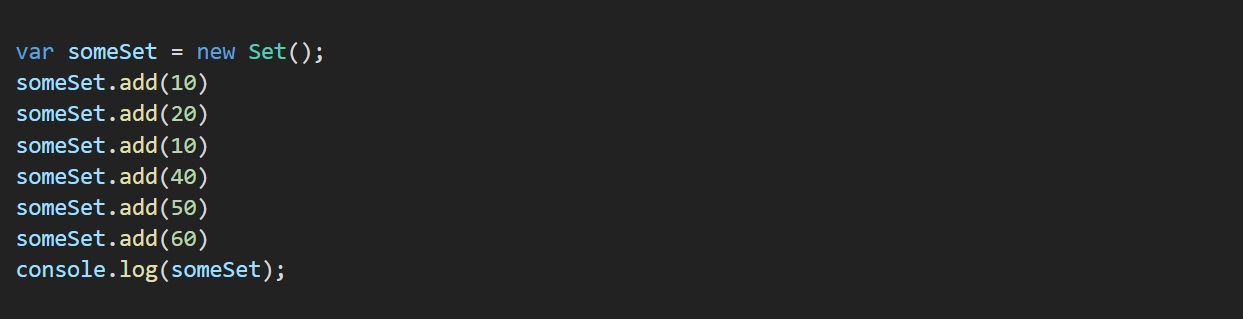As you can see, we first created an empty set, and then we added some elements to the set using the add method. Now, if you observe carefully, we are attempting to add 10 for two times in the set, which is out of the rules for the set. So, we won’t be able to do so. Here is the output –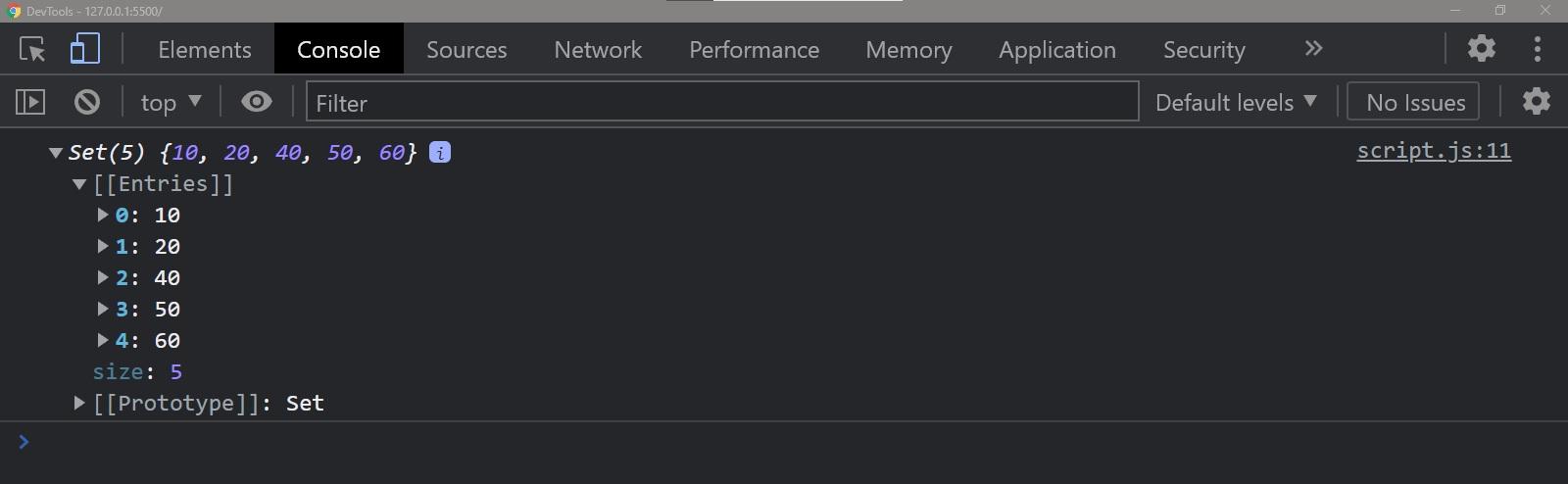As you can see, the 10 was not added again, since it would get repeated then. We have got 5 elements into our set.

Also, we can add different types of data to the set. Here is an example, just so that you understand how can we add different data to the set.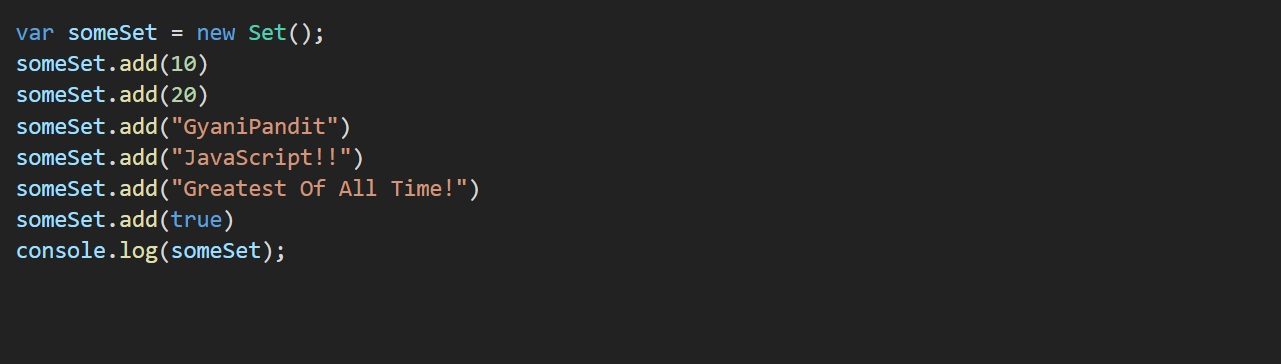As you can see, we are able to add different types of data to the set. This is what the output looks like now –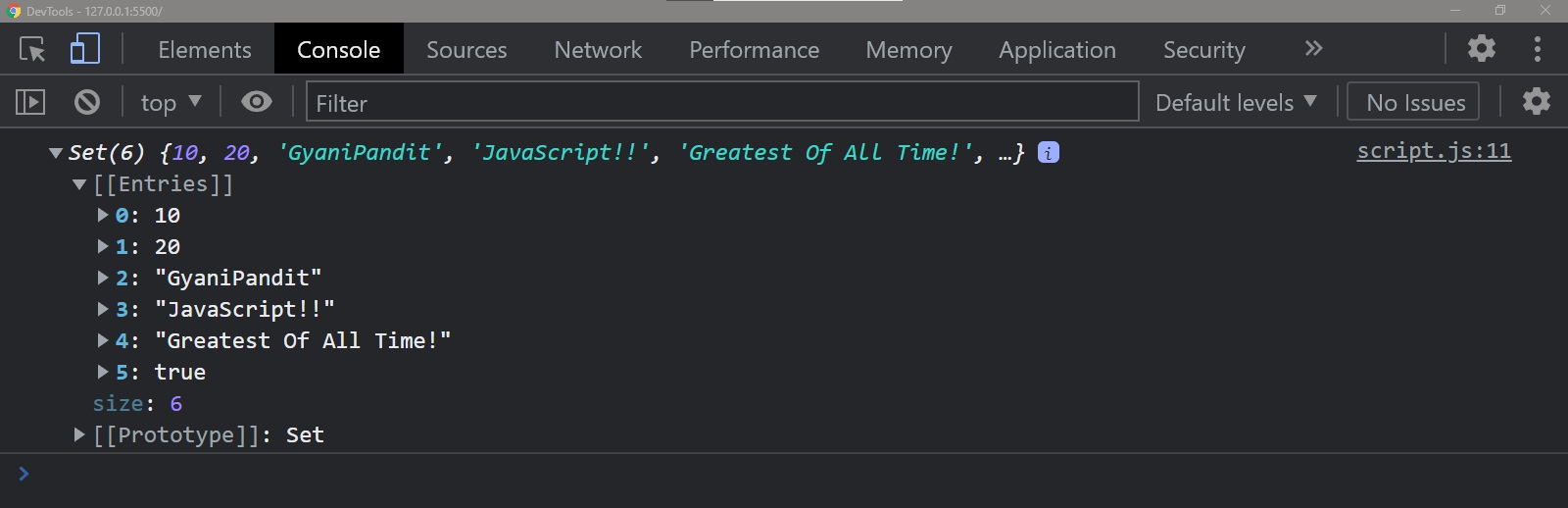So, this states that we can add data of different types to our set.